# Vector calculus- region-density-mass

## Homework Statement

View attachment 229290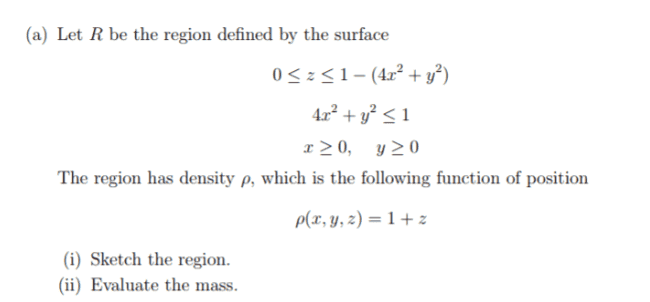## Homework Equations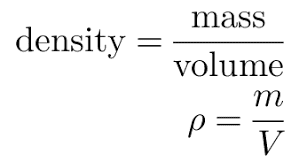## The Attempt at a Solution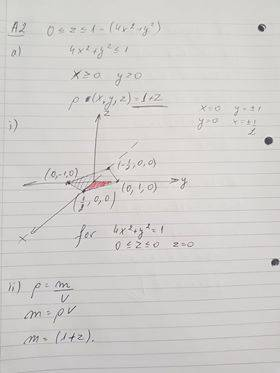I am not sure what equation to use for the volume[/B]

#### Attachments

Chandra Prayaga
The attempt that you posted is too small and I cannot click on it to get a better view. But from what I can see, I suggest the following.
1. Understand, from the ranges of x, y and z given by you, the shape of the region you are interested in. In other words, answer the first question and sketch the region. In your graph, you only showed a few isolated points. You did not sketch the region in space.
2. The relevant equation posted by you is not adequate. Density = mass / volume is good only if the density is constant. In your case, the density depends on position (your equation: ρ = 1 + z). Then you must use the differential form:

dm = ρ dV,

and integrate this to get the total mass. It will be a triple integral.

•Jozefina Gramatikova
The attempt that you posted is too small and I cannot click on it to get a better view. But from what I can see, I suggest the following.
1. Understand, from the ranges of x, y and z given by you, the shape of the region you are interested in. In other words, answer the first question and sketch the region. In your graph, you only showed a few isolated points. You did not sketch the region in space.
2. The relevant equation posted by you is not adequate. Density = mass / volume is good only if the density is constant. In your case, the density depends on position (your equation: ρ = 1 + z). Then you must use the differential form:

dm = ρ dV,

and integrate this to get the total mass. It will be a triple integral.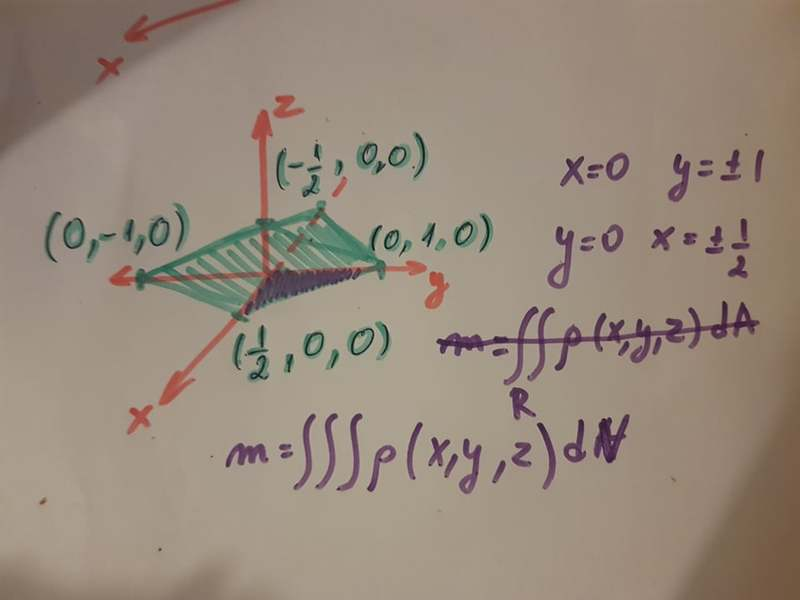I hope you can see the picture better now. I was wondering if I need the whole rectangle as a region or just the purple triangle that I sketched there?

#### Attachments

Last edited:
I guess it is just the triangle, because we have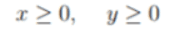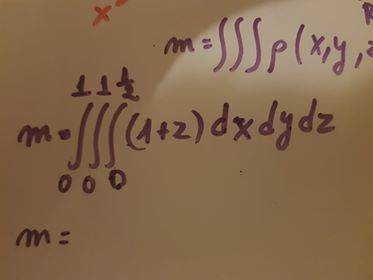Is my final equation ok?

Last edited:
haruspex